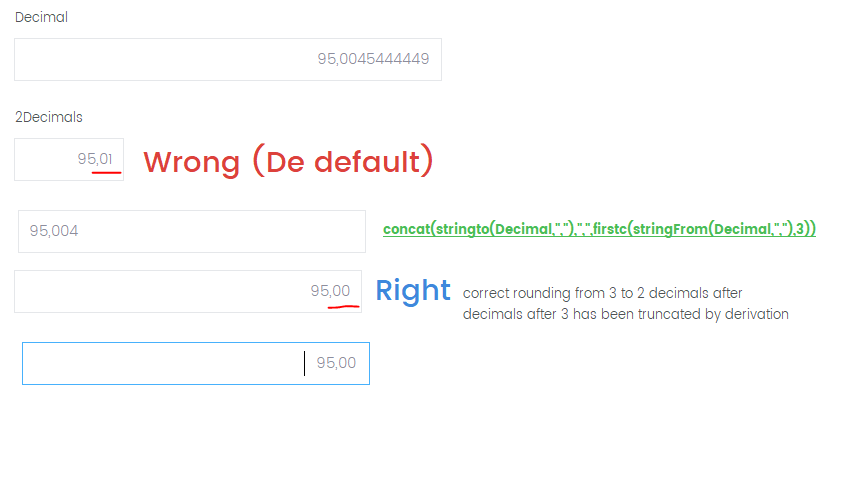Simplicty and flexibility!

Problem with rounding of decimals correctly 95.0045673 returns 94.01

Started by Henk Spierings

Problem with rounding of decimals correctly 95.0045673 returns 94.01

We now have a numberfield( total 12 digits, from which 6 decimals) with the the value 95,004873. Besides this field we have a field with 12 digits and 2 decimals and holds the value 95,01 . If you look at the way of rounding it, it makes no sense.

Can you tel me how dataease is doing this, may be I then can understand these idiot things. It looks like its rounding the figures from right to left.

So the 3 from this example doesnt influence it. The 7 rounds the 8 to 9. The 9 rounds to a 5 and than we have the 95,01. But that is not correct ?

Written by Henk Spierings 22/09/20 at 10:13:20 Dataease [{8}]FIVE

Re:Problem with rounding of decimals correctly 95.0045673 returns 94.01You got it right and from invstigating the code this has been fixed (and broken) a number of times.

The problem is really only there if the 4th decimal is larger than 4, but if it is the entire thing goes haywire. The reason for this is that it was fixed for Money at some stage (2 decimals) and nobody thought about what happens if there is more.

Anyway fix was very "bad" so now we have done proper fix.

But as the fix is only there in a not yet released (as of the day this is written) version of DE9 the fix for now is a workaround.

The workaroud is quite straight forward (I have provided two).

concat(stringto(Decimal,","),",",firstc(stringFrom(Decimal,","),3))

Another ?mistake? in DE is to allow localized decimal separators so if used in England etc. you must check for . rather than , but otherwise it should be the same

What I do is cut out all of the number to , and then I cut out 3 digits of decimal and concat it back up. Then you can round it off.

Another way to do it is:

floor(Decimal*1000)/1000

The reason I would use the former is that if you use the latter you can theoretically run out of digits.

DataEase support 14 digits so in a float you can have 7,7 , 14,0 , 0,14 and any variation between. Same goes for fixed. If you have 7 decimals you can only have 7 digits etc.

So theoretically if you calculate on billions with 7 decimals you might have a problem.

You choose, the latter if of course the most elegant, while the former look much more impressing to an untrained eye ;-)

PS! Problem fixed in 9.0.0.3938 22-09-2020

Written by DataEase 22/09/20 at 12:25:08 Dataease [{8}]FIVE
DG3_ForumList